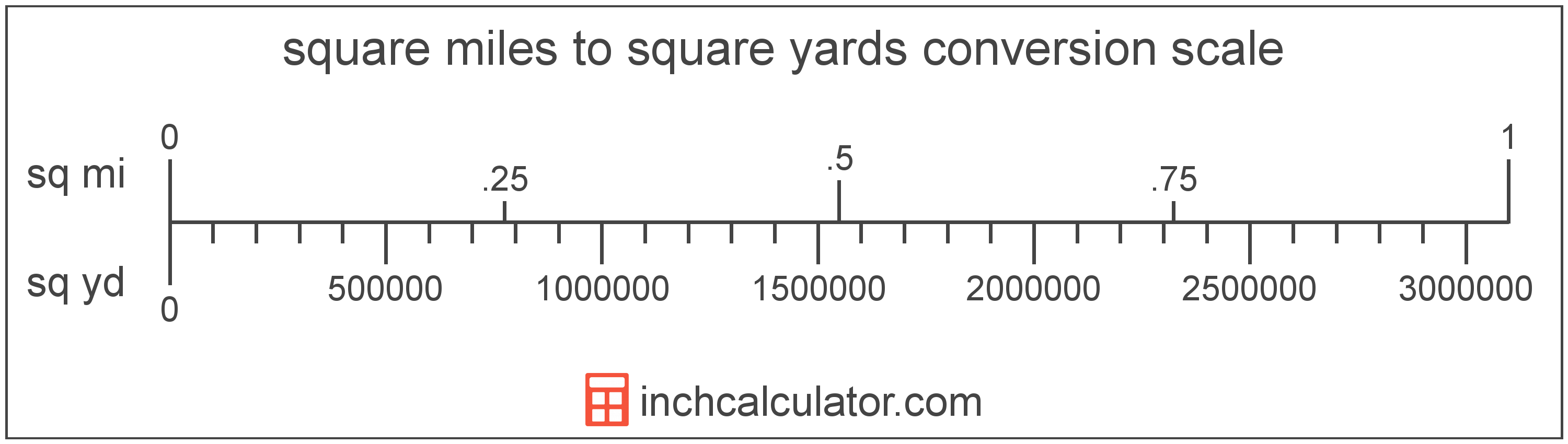# Square Yards to Square Miles Converter

Enter the area in square yards below to get the value converted to square miles.

(find square yards)
Results in Square Miles:1 sq yd = 3.2283E-7 sq mi
Hint: use a scientific notation calculator to convert E notation to decimal

Do you want to convert square miles to square yards?

## How to Convert Square Yards to Square Miles

To convert a measurement in square yards to a measurement in square miles, multiply the area by the following conversion ratio: 3.2283E-7 square miles/square yard.

Since one square yard is equal to 3.2283E-7 square miles, you can use this simple formula to convert:

square miles = square yards × 3.2283E-7

The area in square miles is equal to the area in square yards multiplied by 3.2283E-7.

For example, here's how to convert 5,000,000 square yards to square miles using the formula above.
square miles = (5,000,000 sq yd × 3.2283E-7) = 1.614153 sq mi## What Is a Square Yard?

One square yard is equivalent to the area of a square with sides that are each 1 yard in length. One square yard is roughly equal to 9 square feet or 0.836127 square meters.

The square yard is a US customary and imperial unit of area. A square yard is sometimes also referred to as a square yd. Square yards can be abbreviated as sq yd, and are also sometimes abbreviated as yd². For example, 1 square yard can be written as 1 sq yd or 1 yd².

You can use a square yards calculator to calculate the area of a space if you know its dimensions.

## What Is a Square Mile?

One square mile is equal to the area of a square with sides that are each 1 mile long. One square mile is roughly equal to 2.59 square kilometers or 640 acres.

The square mile is a US customary and imperial unit of area. Square miles can be abbreviated as sq mi, and are also sometimes abbreviated as mi². For example, 1 square mile can be written as 1 sq mi or 1 mi².

## Square Yard to Square Mile Conversion Table

Table showing various square yard measurements converted to square miles.
Square Yards Square Miles
1 sq yd 0.00000032283 sq mi
2 sq yd 0.00000064566 sq mi
3 sq yd 0.00000096849 sq mi
4 sq yd 0.0000012913 sq mi
5 sq yd 0.0000016142 sq mi
6 sq yd 0.000001937 sq mi
7 sq yd 0.0000022598 sq mi
8 sq yd 0.0000025826 sq mi
9 sq yd 0.0000029055 sq mi
10 sq yd 0.0000032283 sq mi
100 sq yd 0.000032283 sq mi
1,000 sq yd 0.000323 sq mi
10,000 sq yd 0.003228 sq mi
100,000 sq yd 0.032283 sq mi
1,000,000 sq yd 0.322831 sq mi
10,000,000 sq yd 3.2283 sq mi